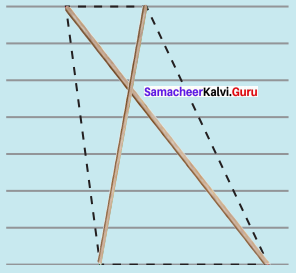Samacheer Kalvi 8th Maths Solutions Term 3 Chapter 3 Geometry Intext Questions

Students can Download Maths Chapter 3 Geometry Intext Questions and Answers, Notes Pdf, Samacheer Kalvi 8th Maths Book Solutions Guide Pdf helps you to revise the complete Tamilnadu State Board New Syllabus and score more marks in your examinations.

Tamilnadu Samacheer Kalvi 8th Maths Solutions Term 3 Chapter 3 Geometry Intext Questions

Exercise 3.1
Think (Text book Page no. 53)

Question 1.
In any acute angled triangle, all three altitudes are inside the triangle. Where will be the orthocentre? In the interior of the triangle or in its exterior?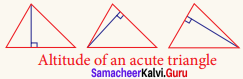Solution:
Interior of the triangle.

Question 2.
In any right angled triangle, the altitude perpendicular to the hypotenuse is inside the triangle; the other two altitudes are the legs of the triangle. Can you identify the orthocentre in this case?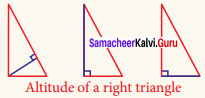Solution:
Vertex containing 90°Question 3.
In any obtuse angled triangle, the altitude connected to the obtuse vertex is inside the triangle, and the two altitudes connected to the acute vertices are outside the triangle. Can you identify the orthocentre in this case?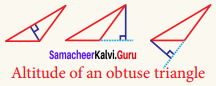Solution:
Exterior of the triangle.

Try These (Text book Page no. 56)

Identify the type of segment required in each triangle:
(median, altitude, perpendicular bisector, angle bisector)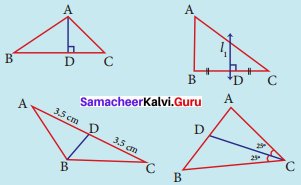(ii) l1 = ………..
(iii) BD = …………
(iv) CD = …………
Solution:
(ii) l1 = Perpendicular bisector
(iii) BD = Median
(iv) CD = Angular bisector

Exercise 3.3
Activity 1. (Text book Page no. 60)

Question 1.
A pair of identical 30°-60°-90° set-squares are needed for this activity. Place them as shown in the figure.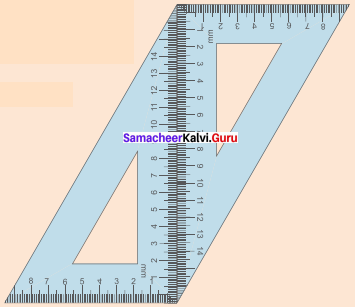1. What is the shape we get? It is a parallelogram.
2. Are the opposite sides parallel?
3. Are the opposite sides equal?
4. Are the diagonals equal?
5. Can you get this shape by using any other pair of identical set-squares?

Solution:

1. It is a parallelogram.
2. Yes
3. Yes
4. no
5. yes

Question 2.
We need a pair of 30°-60°-90° set- squares for this activity. Place them as shown in the figure.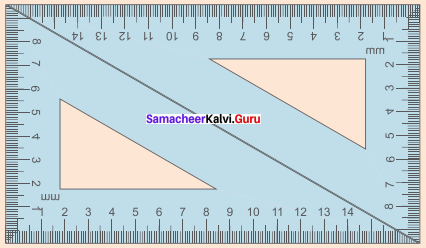(i) What is the shape we get?
(ii) Is it a parallelogram?
It is a quadrilateral; infact it is a rectangle. (How?)
(iii) What can We say about its lengths of sides, angles and diagonals? Discuss and list them out.
Solution:
(i) Rectangle
(ii) Yes, Opposite sides are equal. All angles = 90°
(iii) Opposite sides are equal.
All angles are equal and are = 90°.
Diagonals are equalQuestion 3.
Repeat the above activity, this time with a pair of 45°-450-90° set-squares.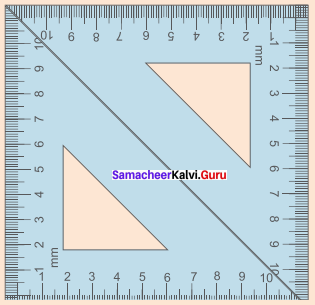(i) How does the figure change now? Is it a parallelogram? It becomes a square! (How did it happen?)
(ii) What can we say about its lengths of sides, angles and diagonals? Discuss and list them out.
(iii) How does it differ from the list we prepared for the rectangle?
Solution:
(i) All sides are equal
(ii) All sides are equal
All angles = 90°
Diagonals equal
(iii) All sides are equal.
Diagonals bisects each other.

Question 4.
We again use four identical 30°-60°-90° set- squares for this activity.
Note carefully how they are placed touching one another.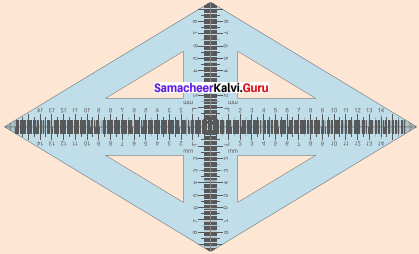(i) Do we get a parallelogram now?
(ii) What can we say about its lengths of sides, angles and diagonals?
(iii) What is special about their diagonals?
Solution:
(i) Yes
(ii) All sides equal.
(iii) Diagonals bisects perpendicularly.

Try These (Text book Page no. 62)

Question 1.
Say True or False:
(a) A square is a special rectangle.
(b) A square is a parallelogram.
(c) A square is a special rhombus.
(d) A rectangle is a parallelogram
Solution:
(a) True
(b) True
(c) True
(d) True

Question 2.
(a) Which have diagonals bisecting each other.
(b) In which the diagonals are perpendicular bisectors of each other.
(c) Which have diagonals of different lengths.
(d) Which have equal diagonals.
(e) Which have parallel opposite sides.
(f) In which opposite angles are equal.
Solution:
(a) Square, rectangle, parallelogram, rhombus.
(b) Rhombus and square.
(c) Parallelogram and Rhombus
(d) Rectangle, square.
(e) Square, Rectangle, Rhombus, parallelogram.
(f) Square, rectangle, rhombus, parallelogramQuestion 3.
Two sticks are placed on a ruled sheet as shown. What figure is formed if the four corners of the sticks are joined?
(a)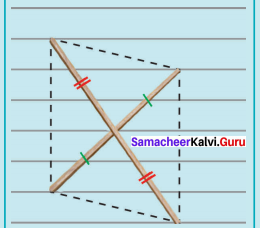Two unequal sticks. Placed such that their midpoints coincide.
Solution:
Parallelogram

(b)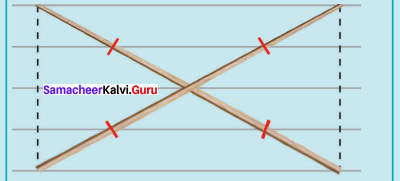Two equal sticks. Placed such that their midpoints coincide.
Solution:
Rectangle

(c)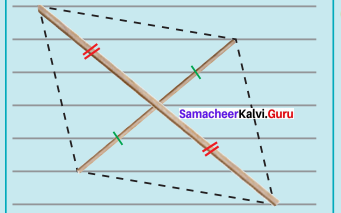Two unequal sticks. Placed intersecting at mid points perpendicularly.
Solution:
Rhombus

(d)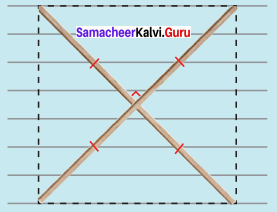Two equal sticks. Placed intersecting at mid points perpendicularly.
Solution:
Square

(e)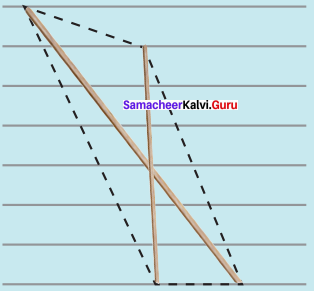Two unequal sticks. Tops are not on the same ruling. Bottoms on the same ruling. Not cutting at the mid point of either.
Solution:
(f)